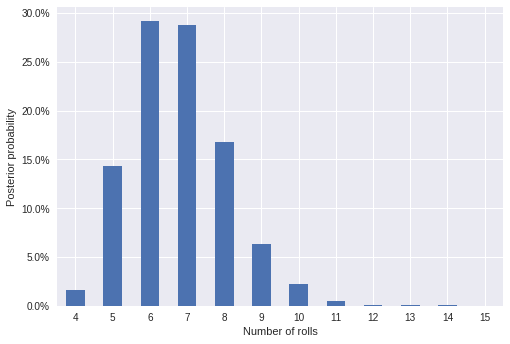In :
%matplotlib inline

In :
from collections import Counter
from tqdm import trange

In :
from matplotlib.ticker import StrMethodFormatter
import numpy as np
import pandas as pd
import seaborn as sns
from scipy import stats

In :
sns.set()
pct_formatter = StrMethodFormatter('{x:.1%}')


We'll use approximate Bayesian computation (ABC) to solve the Game of Ur problem that Allen Downey posed on Twitter recently.

Our observation is that the piece is on the thirteenth square, and we want to infer the posterior distribution of the number of turns it took to get there.

In :
OBS = 13


As with all Bayesian inference, ABC requires both a prior distribution on the number of rolls and a data generation process (likelihood). Since it must take at least four rolls to reach spot 13, we place a $U(4, 40)$ prior on the number of rolls. Note that while the number of rolls needed to reach spot 13 is theoretically unbounded, it would be extremely unlucky not to pass spot 13 within 40 rolls, so we use this truncated uniform prior for computational simplicity.

In :
min_rolls = int(np.ceil(OBS / 4))
prior = stats.randint(min_rolls, 10 * min_rolls)


Each turn results in moving a number of spaces drawn from a $\textrm{Bin}\left(4, \frac{1}{2}\right)$ distribution.

In :
dice = stats.binom(4, 0.5)

def simulate_spot(rolls, dice):
return dice.rvs(size=rolls).sum()


The simplest version of ABC generates posterior samples by the following algorithm.

1. Generate a sample from the prior.
2. Simulate the data generating process given the prior sample.
3. If the simulated data matches the observed data, save the sample. If not, repeat the above process.

The following function implements this algorithm.

In :
def generate_abc_sample(obs, prior, dice):
while True:
rolls = prior.rvs()
spot = simulate_spot(rolls, dice)

if spot == obs:
return rolls


We now generate 5,000 samples from the posterior using ABC.

In :
N_SAMPLES = 5000

In :
post_sample_counts = Counter(generate_abc_sample(OBS, prior, dice) for _ in trange(N_SAMPLES))

100%|██████████| 5000/5000 [01:09<00:00, 72.00it/s]

In :
post_df = (pd.DataFrame.from_dict(post_sample_counts, orient='index')
.rename(columns={0: 'samples'})
.sort_index())

In :
post_df.head()

Out:
samples
4 79
5 716
6 1458
7 1440
8 841

Below we visualize the posterior distribution of these samples. The fact that the largest observed sample is much smaller than the point at which we truncated our prior (40) is circumstantial evidence that truncation has not had too large an effect.

In :
ax = (post_df.div(N_SAMPLES)
.plot(kind='bar', legend=False, rot=0))

ax.set_xlabel("Number of rolls");

ax.yaxis.set_major_formatter(pct_formatter);
ax.set_ylabel("Posterior probability");